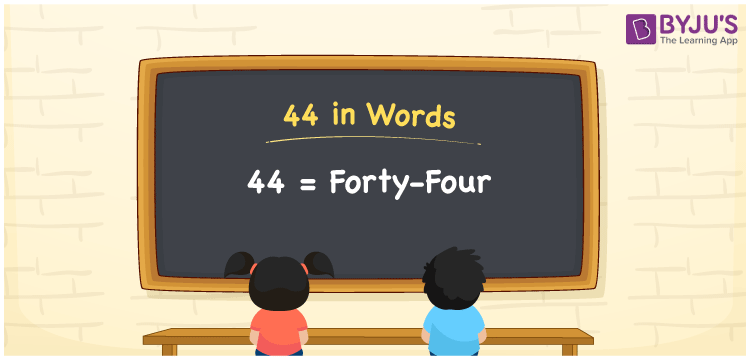# 44 in words

44 in words is written as Forty Four. 44 represents the count or value. The article on Counting Numbers can give you an idea about count or counting. The number 44 has 1, 2, 4, 11, 22 and 44 as factors. It is used at multiple places and instances. For example, “ I have 44 days before my summer vacation.” Another example, “The cricketer scored Forty four runs in yesterday’s match”.

 44 in words Forty Four Forty Four in Numbers 44

## 44 in English Words## How to Write 44 in Words?

We can convert 44 to words using a place value chart. The number 44 has 2 digits. Let us check how 44 would look like in a chart that shows the place value up to 2 digits.

 Tens Ones 4 4

Thus, we can write the expanded form as:

4 × Ten + 4 × One

= 4 × 10 + 4 × 1

= 44

= Forty Four.

44 is the natural number that is succeeded by 43 and preceded by 45.

44 in words – Forty Four.

Is 44 an odd number? – No.

Is 44 an even number? – Yes.

Is 44 a perfect square number? – No.

Is 44 a perfect cube number? – No.

Is 44 a prime number? – No.

Is 44 a composite number? – Yes.

## Solved Example

1. Write the number 44 in expanded form

Solution: 4 x 10 + 4 x 1

We can write 44 = 40 + 4

= 4 x 10 + 4 x 1

## Frequently Asked Questions on 44 in words

Q1

### How to write 44 in words?

44 in words is written as Forty Four.
Q2

### State True or False. 44 is an even number and hence it is divisible by 2?

True. As 44 is an even number, it is divisible by 2. It is also divisible by 4.
Q3

### Is 44 a perfect square number?

No. 44 is not a perfect square number